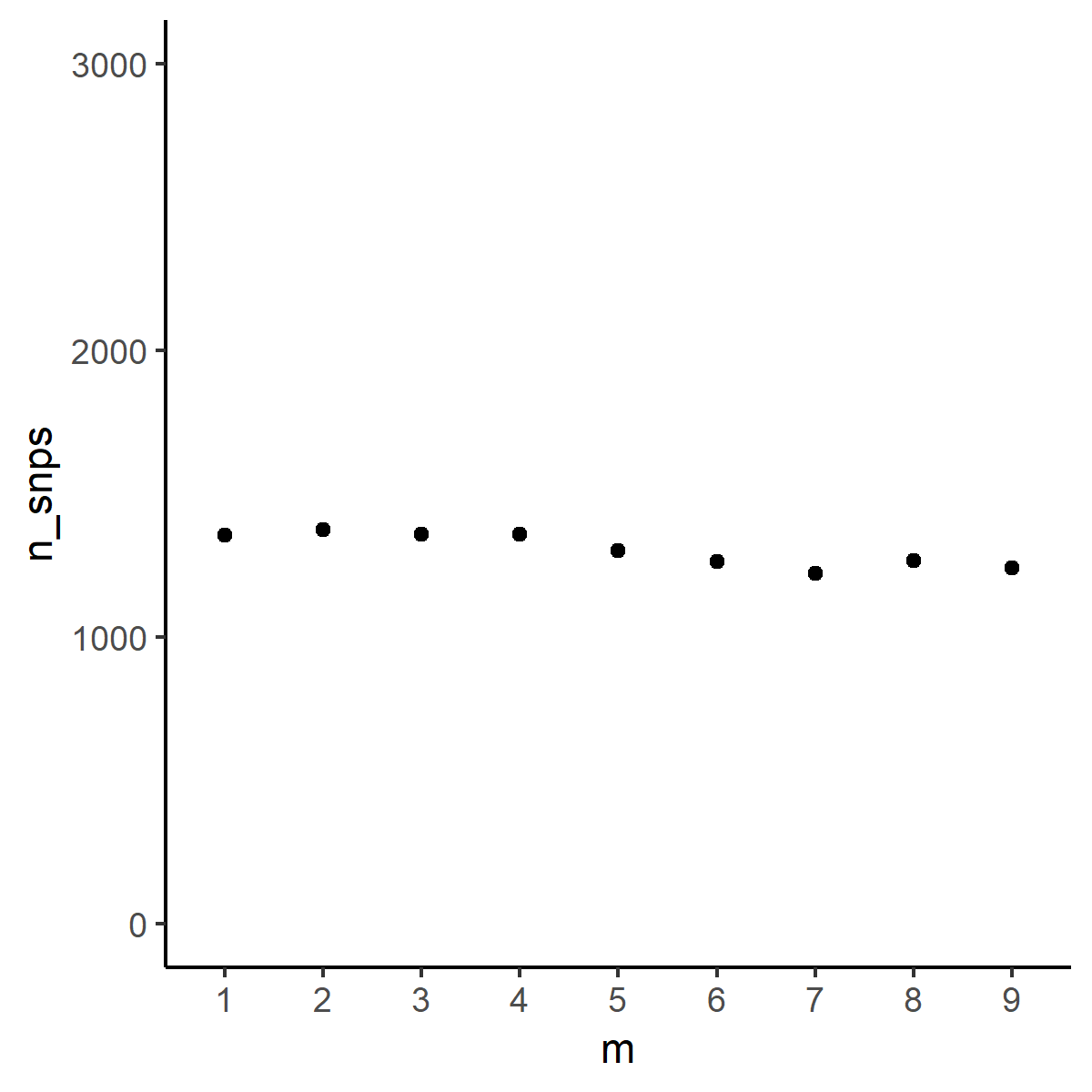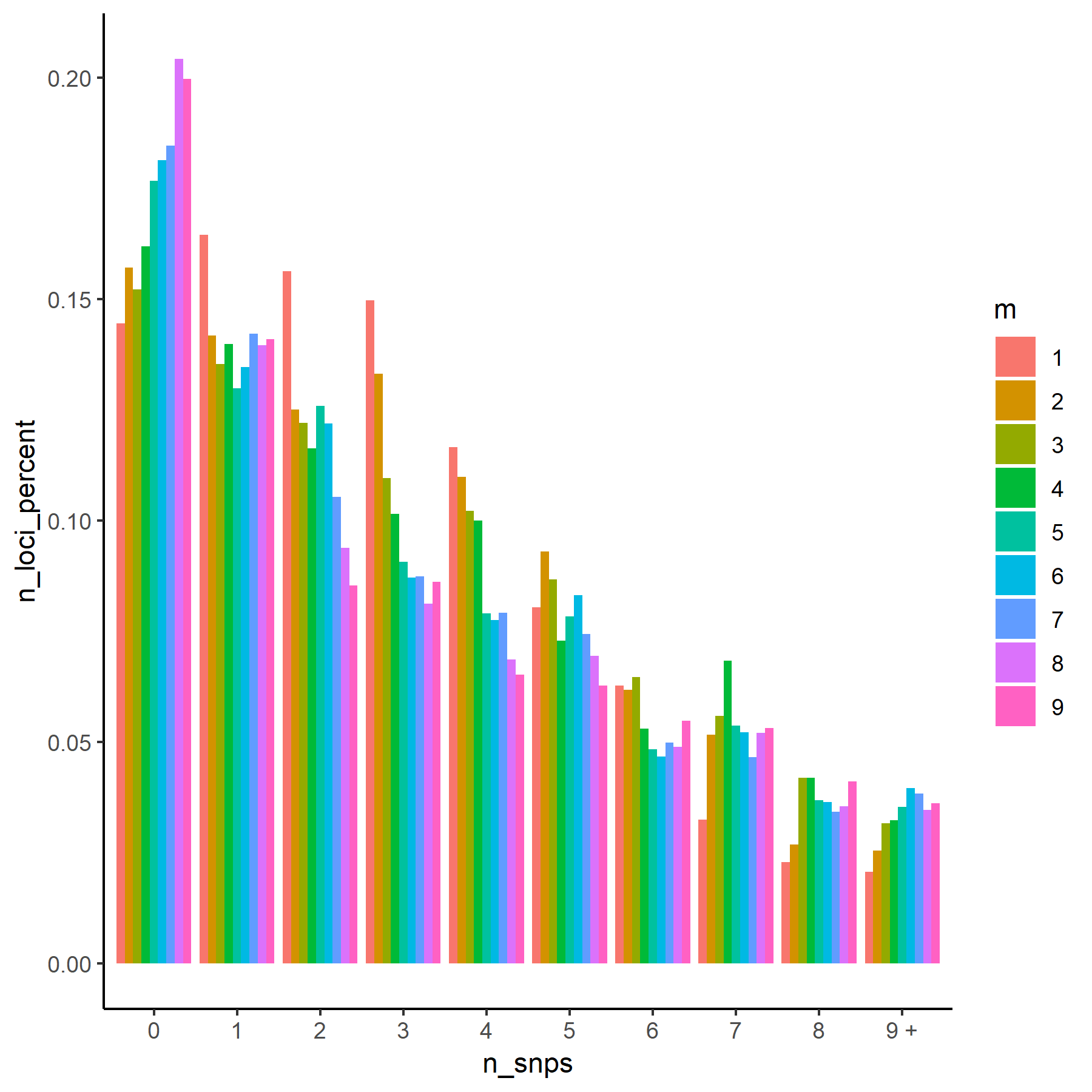Want to share your content on R-bloggers? click here if you have a blog, or here if you don't.

The recommended way to figure out the best parameters to use in stacks for denovo RAD-seq analysis is to test parameter combinations for M and n. (Paris et al. 2017, Rochette and Catchen 2017).

The authors of stacks recommend testing M and n from 1 to 9 and then visualizing the distribution of loci against the number of SNPs on the loci.From Rochette and Catchen 2017

stacks provides a script to extract distributions using `stacks-dist-extract` but you will have to run it repeatedly by hand for every parameter.

Here is a bash script with a loop to extract the distribution of SNPs for each parameter combination and a R script to visualize the distributions in R similar to Rocheete and Catchen’s figure.

In this script, it is assumed that folder names follow Rochette and Catchen’s protocol (stacks.M1, stacks.M2 etc)

```Home
+-- stacks.M1
|   +-- populations.r80
|   +-- populations.log
|   +-- populations.log.distribs
|   +-- ind.1.alleles.tsv.gz
|   +-- ind.1.matches.bam
|   +-- ind.1.snps.tsv.gz
|   +-- ind.1.tags.tsv.gz
|   ...
|-- stacks.M2
|-- stacks.M3
|-- stacks.M4
...
#!/bin/bash

# load stacks for those who are on clusters, this is for SLURM
# change the numbers in the next line to match the directory values
for i in 1 2 3 4 5 6 7 8 9
do
stacks-dist-extract stacks.M\${i}/populations.r80/populations.log.distribs snps_per_loc_postfilters > \${i}_snp_distribution.tsv
done```

There is also a pure `awk` solution not involving stacks scripts.

```#!/bin/bash

# load stacks for those who are on clusters, this is for SLURM

for i in 1 2 3 4 5 6 7 8 9
do
awk '/snps_per_loc_postfilters/{flag=1; next} /END/{flag=0} flag' stacks.M\${i}/populations.r80/populations.log.distribs > \${i}_snp_distribution.tsv
done

```

Then in R, make a data frame combining all parameter distributions before visualizaing in `ggplot2`. Here, the data comes from a GBS analysis of garlic mustard (Alliaria petiolata).

```count <- 1
files <- list.files(path = "", pattern="*_snp_distribution.tsv", full.names = T)
for (i in files[1:9]){
table\$n_loci_percent<- table\$n_loci/sum(table\$n_loci)
table\$m<- count
write.table(table, "distributions.tsv", append=T, row.names=F, col.names = F)
snp_count <- data.frame("m"= count, "n_snps"=sum(table\$n_loci))
write.table(snp_count, "total_count.tsv", append=T, row.names=F, col.names = F)
count <- count + 1
}```

`total_count.tsv` is used to display total SNP by parameter.

```library(ggplot2)
names(snp_count)<-c("m", "n_snps")
snp_count\$m<-as.factor(snp_count\$m)
p<-ggplot(data=snp_count, aes(x=m, y=n_snps)) +
geom_point() + theme_classic() + scale_y_continuous(limits = c(0, 3000))
p```Scatterplot of parameter and total SNP count

`distributions.tsv` is used to show parameter distributions.

```snp_table<-read.delim("distributions.tsv", sep=" ", header=F)
names(snp_table)<- c("n_snps","n_loci", "n_loci_percent", "m")
snp_table\$n_loci_percent<-snp_table\$n_loci_percent*100
snp_table\$n_snps<-ifelse(snp_table\$n_snps < 9, snp_table\$n_snps, "9 +")
snp_table\$n_snps<-as.factor(snp_table\$n_snps)
snp_table\$m<-as.factor(snp_table\$m)

q<-ggplot(data = snp_table) +
geom_col(aes(x=n_snps, y=n_loci_percent, fill=m), position="dodge") + theme_classic()
q```Histogram of number of SNPs per locus